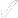PE technical guidance# What is SDR pipe and how does it influence the pressure rating of the pipe?

Quick access

## SDR pipe : "Standard Dimensional Ratio"

The SDR pipe is the "Standard Dimensional Ratio" and refers to the geometry of the pipe. SDR is defined as the ratio of the nominal outside diameter to the nominal wall thickness.

SDR = dn/en

Where dn is the nominal outside diameter of the pipe and en is the nominal (minimum) wall thickness of the pipe.

Therefore a higher SDR indicates a thinner-walled pipe at any given diameter.

The relationship between the SDR and the pressure rating is given by Lames formula for the hoop stress in thick wall cylinders:

s = P(dn - en) / 2 en

This can be rearranged as;

s = P (SDR - 1) / 2

Where s is the maximum hoop stress P is the internal pipe pressure.

The hoop stress is the design stress for the material, which is the (MRS) divided by the overall service (design) coefficient C.

MRS/C = P (SDR - 1) / 2

or rearranging;

P = 2 MRS / C (SDR - 1)

This pressure ‘P' is then defined as the ‘Maximum Operating Pressure' MOP, or the pressure rating of the pipe.

MOP = 2 MRS / C (SDR - 1)

Where MRS and MOP are in MPa

Or

MOP = 20 MRS / C (SDR - 1)

Where MRS is in MPa and MOP is in bar.

## Example : What is the MOP or pressure rating of an SDR11 PE100 water pipe?

For PE100 the MRS = 10 MPa. For water applications the minimum recommended service design coefficient ‘C' is 1.25.

Hence :

MOP = 20 * 10 / 1.25 (11 - 1)

MOP = 16 bar

The following Table shows the range of available SDRs in PE100 pipe.

 SDR Available to Order Readily Commercially Available 7.491113.61717.621263341Last update Thursday 31 May 2018
You may also be interested in
SDR - MOPThursday 07 February 2013

How to use the calculator ? Select the External pipe diameter, MRS, C and either the SDR OR a value for the MOP. If you select the SDR, the MOP is calculated or if you enter a MOP value, the SDR is...

TOP